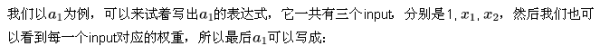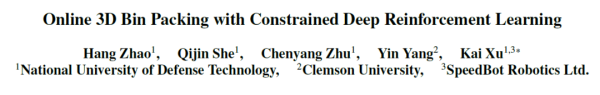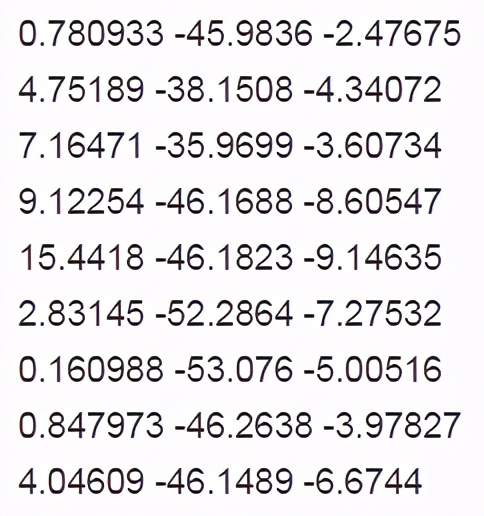# 神经网络与感知机的不同 那么它们之间有什么区别呢?

2020-11-30 14:57:54    来源：51ctoSigmoid我们已经很熟悉了，如果我们把LR模型也看成是一个单层的神经网络的话，那么Sigmoid就是它的激活函数。Sigmoid应用在二分类场景当中单个的输出节点上，输出的值如果大于0.5表示为真，否则为假。在一些概率预估场景当中，也可以认为输出值就代表了事件发生的概率。

importnumpyasnpdefrelu(x):returnnp.where(x>0,x,0)defsigmoid(x):return1/(1+np.exp(-x))classNeuralNetwork():def__init__(self):self.params={}self.params['W1']=np.random.rand(2,3)self.params['b1']=np.random.rand(1,3)self.params['W2']=np.random.rand(3,2)self.params['b2']=np.random.rand(1,2)self.params['W3']=np.random.rand(2,1)self.params['b3']=np.random.rand(1,1)defforward(self,x):a1=np.dot(x,self.params['W1'])+self.params['b1']z1=relu(a1)a2=np.dot(z1,self.params['W2'])+self.params['b2']z2=relu(a2)a3=np.dot(z2,self.params['W3'])+self.params['b3']returnnp.where(sigmoid(a3)>0.5,1,0)if__name__=="__main__":nn=NeuralNetwork()print(nn.forward(np.array([3,2])))

### 推荐文章#### 苹果在AR和VR努力当中又获得了一项新专利#### 强化学习与3D视觉结合新突破：深度强化学习算法与启发式算法的性能对比#### 日本首战告捷，GA成为日本市场上首个规模化上线VR看房企业#### VR / AR仍面临着性能方面的较大考验#### 3D 视觉几乎无所不能计算机如何「看」这个三维世界#### 全家最新推出的一个摆货机器人？！5公里外VR操控，延迟不到一秒#### 聚焦：索尼或于6月初公布PlayStation 5的外形设计